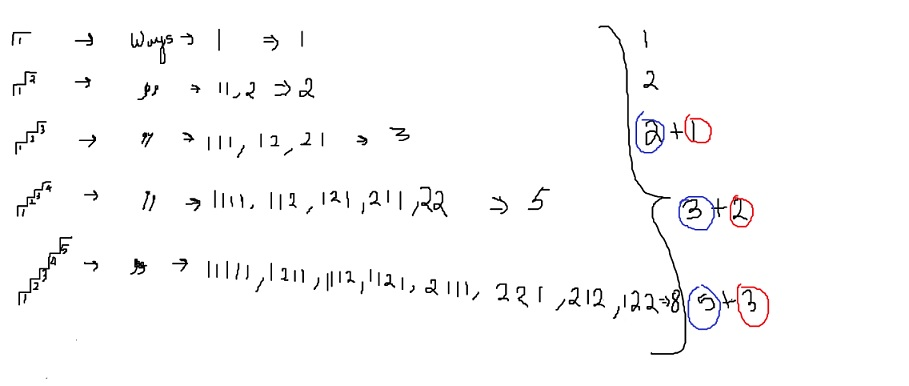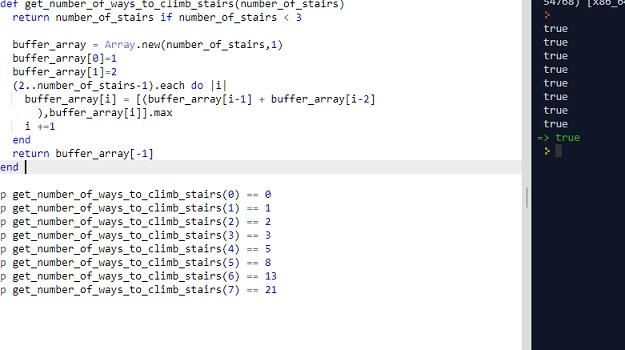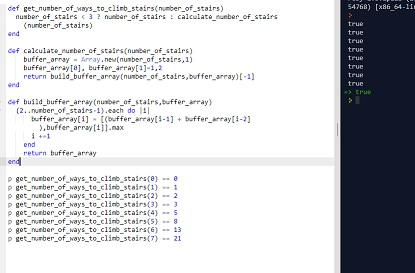# CLEAN CODE: DYNAMIC PROGRAMMING: FIND NUMBERS OF WAYS TO CLIMB STAIRS

By: Saurav

2017-11-11 22:30:00 UTC

You are climbing a stair case. It takes n steps to reach to the top.

Each time you can either climb 1 or 2 steps. In how many distinct ways can you climb to the top?

Example :

Input : 3
Return : 3

Steps : [1 1 1], [1 2], [2 1]

InterviewBit

This problem looks tough and feels like where to start but its actually very simple if you follow a simple strategy to solve problems:

Divide and conquer!!

Lets solve the problem for very small number of stairs and we will use memoization techniques to solve the next problem. You will see this is just a Fibonacci sequence.

I have tried to visualize what pattern I saw in the figure below:As indicated, each ways for given step number (after 2 steps), is the sum of the last two ways.

If you think about combinations, you will feel it makes sense:

Suppose we are given: 1 and (11,2) and I want to know number of combinations possible by combining them:

Number of combinations possible = 11(1), 2(1), (1)2.

Lets write some tests first:

```p get_number_of_ways_to_climb_stairs(0) == 0
p get_number_of_ways_to_climb_stairs(1) == 1
p get_number_of_ways_to_climb_stairs(2) == 2
p get_number_of_ways_to_climb_stairs(3) == 3
p get_number_of_ways_to_climb_stairs(4) == 5
p get_number_of_ways_to_climb_stairs(5) == 8
p get_number_of_ways_to_climb_stairs(6) == 13
p get_number_of_ways_to_climb_stairs(7) == 21```

Attempt 1:

```def get_number_of_ways_to_climb_stairs(number_of_stairs)
return number_of_stairs if number_of_stairs < 3

buffer_array = Array.new(number_of_stairs,1)
buffer_array=1
buffer_array=2
(2..number_of_stairs-1).each do |i|
buffer_array[i] = [(buffer_array[i-1] + buffer_array[i-2]),buffer_array[i]].max
i +=1
end
return buffer_array[-1]
end ```All our tests pass.

Its time to refactor our code, make it clean and let one function do just one thing best.

```def get_number_of_ways_to_climb_stairs(number_of_stairs)
number_of_stairs < 3 ? number_of_stairs : calculate_number_of_stairs(number_of_stairs)
end

#private
def calculate_number_of_stairs(number_of_stairs)
buffer_array = Array.new(number_of_stairs,1)
buffer_array, buffer_array=1,2
return build_buffer_array(number_of_stairs,buffer_array)[-1]
end

def build_buffer_array(number_of_stairs,buffer_array)
(2..number_of_stairs-1).each do |i|
buffer_array[i] = [(buffer_array[i-1] + buffer_array[i-2]),buffer_array[i]].max
i +=1
end
return buffer_array
end```All our tests pass and each function does one thing and just one thing best.

### Let me know what you think! twitter: sprakash24octlinkedin

Owned & Maintained by Saurav Prakash

If you like what you see, you can help me cover server costs or buy me a cup of coffee though donation :)